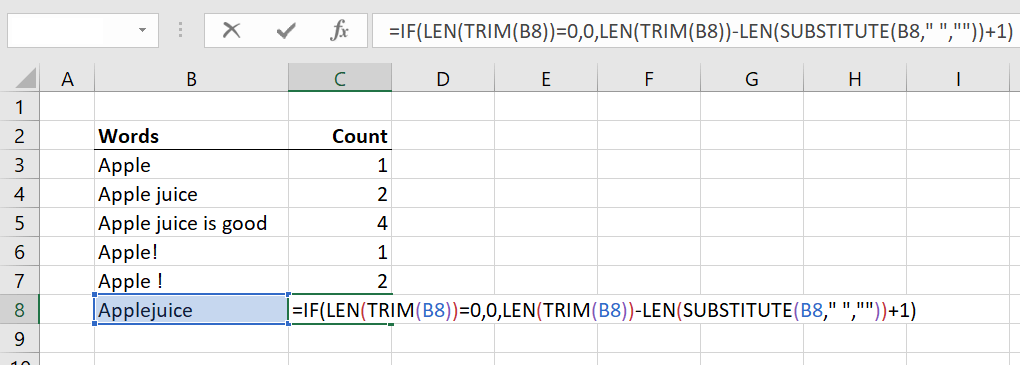# Count Words in Excel

Use the formula to count words

## How to Count Words in Excel

This guide will teach you how to count words in Excel with step-by-step instruction and screenshots. If you’re a fan of the word counting function in MS Word, you will, unfortunately, be disappointed to know that standard Excel Functions do not have this same feature. There is, however, a special formula you can use to count the words in a single cell, or a selection of cells. This guide will show you how, step by step.

### Excel Word Count Formula

Below is the Excel word count formula you can use to determine how many words are contained in a cell:

=IF(LEN(TRIM(cell))=0,0,LEN(TRIM(cell))-LEN(SUBSTITUTE(cell,” “,””))+1)

By using a combination of LEN, TRIM and SUBSTITUTE formulas you can get Excel to count up the number of words for you.

Let’s review what each of these formulas does:

• LEN = returns the length of a string (i.e., tells you all the characters including spaces in a cell)
• TRIM = removes all spaces from a string of text except for spaces between words
• SUBSTITUTE = replaces all instances of something in a cell (in this case replaces ” ” all spaces with no space””)

So, how does it actually work?

The formula just takes the difference between (1) the length of characters in the cell with spaces and (2) the length of characters in the cell without spaces.  The difference, plus one, must be equal to the number of words in the cell.

### Count Words in Excel Example

Let’s look at an example of how to count words in Excel by putting the formula into action. Below is a list of cells that contain various combinations of words, sentences, and characters. Let’s see how the formula counts the words.As you can see above, the formula is able to determine the number of words in each cell.

Here is an explanation for each example:

• Cells B3, B4, and B5 are all “normal” word counts
• Cell B6 shows that the “!” is not counted as long as there is no space before it
• Cell B7 indicated that a symbol such as “!” will count as a word if it has space before it
• Cell B8 shows that if there is no space between the words, the two words will count as one

Thank you for reading CFI’s guide on how to count words in Excel, and the formula you can use to do so with. CFI is a leading provider of Excel Training Courses that help financial analysts become world-class at their jobs.

To continue learning, these guides will also be helpful:

• Index Match Formula
• VLOOKUP Guide
• If Statement Between Numbers